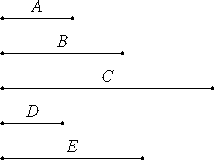# Proposition 30

If two numbers, multiplied by one another make some number, and any prime number measures the product, then it also measures one of the original numbers.

Let the two numbers A and B multiplied by one another make C, and let any prime number D measure C.

I say that D measures one of the numbers A or B.Let it not measure A.

VII.29

Now D is prime, therefore A and D are relatively prime.

Let as many units be in E as the times that D measures C.

VII.Def.15

Since then D measures C according to the units in E, therefore D multiplied by E makes C.

Further, A multiplied by B also makes C, therefore the product of D and E equals the product of A and B.

VII.19

Therefore D is to A as B is to E.

But D and A are relatively prime, relatively prime numbers are also least, and the least measure the numbers which have the same ratio the same number of times, the greater the greater and the less the less, that is, the antecedent the antecedent and the consequent the consequent, therefore D measures B.

Similarly we can also show that, if D does not measure B, then it measures A. Therefore D measures one of the numbers A or B.

Therefore, if two numbers, multiplied by one another make some number, and any prime number measures the product, then it also measures one of the original numbers.

Q.E.D.

## Guide

This proposition states that if p is a prime number, then whenever p divides a product of two numbers, then it divides at least one of them. This is actually a property that characterizes prime numbers, that is to say, no composite number has this property. (For if c is a composite number, c = ab, so c divides the product ab but it doesn’t divide either factor a or b.)

#### Outline of the proof

Assume that a prime number d divides the product ab.

The form of the proof is interesting. Euclid shows that if d doesn’t divide a, then d does divide b, and similarly, if d doesn’t divide b, then d does divide a. Therefore, it divides either one or the other.

Suppose d does not divide a. Then, by VII.29, d is relatively prime to a. Let e be the number ab/d. Then d : a = b : e. By VII.21, the ratio d : a is in lowest terms, and so, by VII.20, d divides b.

#### Use of this proposition

This proposition is used in IX.14.